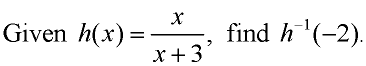MathBits.com presents ...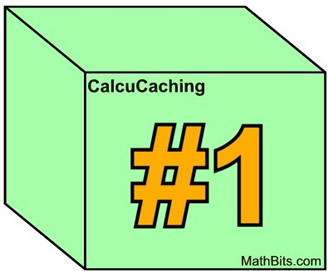Welcome to the Differential "CalcuCaching"
version of MathCaching!

(This level is for Calculus students.)

You are searching for 10 hidden internet boxes. You have just found the first box. Clues to finding the remaining boxes depend upon your ability to solve Calculus problems.
Good luck!

Solve the following 5 problems:

 1. Given:Which of the numbered choices is the domain of f (x)? 1) All Reals 2) All Reals excluding -1 and 5 3) All Reals excluding 1 and -5 4)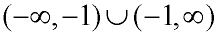5)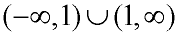2. Which of the following functions is/are odd function(s)? 1)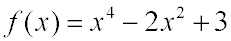2)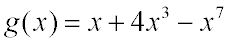3)4) Both 1 and 2 5) Both 1 and 3     3. If, then h (x) = 1)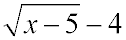2)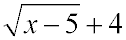3)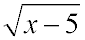4)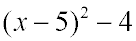5)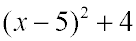4. Given f (x) = 2x2 - x + 12 and g(x) = 5 - x, find the value of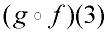.       5.